# Can you help me to understand Op Amps?

#### JosXD

Joined Mar 16, 2022
33
Hi,

First of all I'm not a circuit/electrical engineer, I'm a software developer, even tho I learned to desing pcb my knowledge is very basic( battery,resistors,leds), so If you notice I write non sense things you will know why. What I'm doing here? well kind of a hobby.

I've been watching and watching videos about Op Amps and I feel there is a thing I would like to be explained more deeply, I'm going to post 2 pictures, 1 of open loop and another of feedback resistor.

Open loop: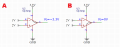In the above diagram there are two cases, one video said that A) If No-inverting input(+) > inverting input(-) (2>1) then positive source is shorted to output Vo=3.3v and B) if no-inverting(+) < inverting (-) (1<2) then gnd or negative souce is shorted to output Vo=0v.

But there is a thing that the video didn't mention and others yes, inverting and no-inverting will have the same Voltage, the question is how? let's take the A) option, after V+ being shorted to Vo the amplifier decreases no-inverting to 1v to equals the inverting, or put both at 0v?

Feedback Resistor: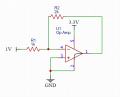This example costs more to understand, inverting input = 1v and no-inverting input = 0v, how does it flows? first the Op Amp notices inverting > no-inverting then shorts gnd(v-) source to Vo, then Op amp makes inverting input to be 0v to match the no-inverting, but what does the feedback resistor( well the videos says it controls the gain ), but does it flows from R1 to Vo or from Vo to R1?

I could think if flows from R1 to Vo beucase of the 1v input > Vo=0v, it confuses me because both should be 0v. Also could think R1 is to limit current for the Op Amp but that's not the case because the internal resistance in the Op Amp inputs are way to higher that it makes current to be too small that can be considered 0.

I know there are formulas, but I'd like to know if there is a grapich way to explain it easier the part of the feedback resistor when -V = 0v or less.

Last edited:

#### Papabravo

Joined Feb 24, 2006
20,385
Your intuitive sense of open loop operation is flawed. The open loop gain of an opamp is quite large in the range of 100,000. This means that if the inverting (-) and non-inverting inputs are different by as little as 1mV, the output will be:
1. 100,000 * (+1mV) = 100V.
2. 100,000 * (-1mV) = -100 V.
Now of course the output has no ability to go to those extremes because it is limited to the power supply range of [0,...,3.3V]. You need a much smaller difference between the inverting (-) input and the non-inverting (+) input to get an output value that is within the power supply range. The practical implications of this are that there are only a few practical applications of opamps in the open loop configuration. Yes, they can be used as comparators, but there are special devices for that purpose that are better suited for that application.

The simplest "closed-loop" application is the voltage follower where you take the output and loop it back to the inverting input. Whatever the non-inverting (+) input now does the output adjusts itself so that the difference between the two inputs is so close to zero as to not be worth mentioning.

Wrap your head around what I have said, and we'll move on to the examples with resistors.

#### MisterBill2

Joined Jan 23, 2018
15,654
First, that video is guilty of a vast oversimplification that is also rather incorrect. I would not trust anything of it's message after that claim about shorting.
"OPAMP" is short for "Operational Amplifier, and in simplest terms the Operation is multiplication of the input signal, which is the difference voltage between the inverting (-) input and the non-inverting (+) input. There are a lot of additional considerations, but that is the description of an Ideal OPAmp., Post #2 continues the description of an "Ideal Op Amp"" .
A graphical description would be a challenge for me to create.

•JosXD

#### JosXD

Joined Mar 16, 2022
33
The simplest "closed-loop" application is the voltage follower where you take the output and loop it back to the inverting input.

But if the output is 0v(GND) and the suply connected to inverting input is 1v, how does output loop to inverting input if 1v>0v, shouldn't current accross R1 move to R2 and then to output?

Now of course the output has no ability to go to those extremes because it is limited to the power supply range of [0,...,3.3V].
Yes yesterday while watching videos, they were making a lot of emphasis of the huge gain of houndred of thousands, and I was like well if the Op Amp has de ability to do that by itself is going to blow lot of stuff, 'till I watched a video where the guy used 2 9v batteries, 5v in inverting input and a gain of 2, he got like -7v in the output.

#### JosXD

Joined Mar 16, 2022
33
First, that video is guilty of a vast oversimplification that is also rather incorrect. I would not trust anything of it's message after that claim about shorting.

A graphical description would be a challenge for me to create.

It's kind of confuse, because some videos say a thing different than others, will keep looking 'till I understand.

#### Papabravo

Joined Feb 24, 2006
20,385
Here is the open loop transfer characteristic of a typical low voltage single supply opamp. Notice the output voltages do not go all the way to the power supply rails.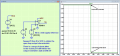Thea actual width of the linear range is actually much smaller than we estimated from the zoomed-out view. It is more like 10µV wide.

If we zoom in on the transition, we get the following: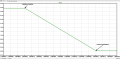The slope of the line tells us the open loop gain which is approximately 110 dB.

Last edited:
•JosXD

#### Papabravo

Joined Feb 24, 2006
20,385
With single supply circuit it is common to bias the amplifier at the mid-point of the supply range. This just means that you hold on of the inputs at a fixed voltage, In my example I chose VCC/2 =1.65 volts. If the inverting input is below the bias voltage value, the output will be as close to the positive supply rail as it can get. In this case we are withing 50 mV of the positive rail. We are also withing 50 mV of ground for th case where the inverting (-) input is above the bias voltage. It looks like the transition starts about 29 µV past the midpoint of the supply.

#### MrChips

Joined Oct 2, 2009
29,230
Don't believe everything you see in a YouTube video or even anything on the web.
An op-amp is an amplifier with very high voltage gain. It does not create shorts to the power supply rails, Vcc or GND.

Vout = voltage gain x (non-inverting input voltage - inverting input voltage)

A typical open loop voltage gain is 100,000.
We don't often use an op-amp with such high gain. We use negative feedback to control the gain to a respectable 1-100 range.

•JosXD

#### panic mode

Joined Oct 10, 2011
2,504
gain is huge (or infinite), inputs are high impedance (infinite) so no current is flowing in or out of inputs voltage difference between inputs is zero unless circuit is out of linear range (saturation).

when there is a feedback, OpAmp will do what ever it takes to get back to the no-difference between inputs.

in your unnamed circuit with feedback ("C"?), one input is at 0V. so the other is supposed to be also at 0V (or try to get there).
this means current through R1 is 1mA. but since input impedance is high, the only way for current is through R2 to output. to keep the input at 0V (to match other input), output would need to go to -2V. but... that is impossible with that supply - any point in the circuit can only be between 0V and 3.3V. so output will try to go to -2V but the lowest it can get to is 0V. so the current from 1V (before R1) will not really be 1mA, it will be lower since (1V-0V)/(R1+R2)=1V/3k=0.33mA and the input is not really at 0V, more like 0.66V.

Last edited:
•JosXD

#### JosXD

Joined Mar 16, 2022
33
Here is the open loop transfer characteristic of a typical low voltage single supply opamp. Notice the output voltages do not go all the way to the power supply rails.
Thank you Papabravo!

I'll take a close look at it.

#### MrChips

Joined Oct 2, 2009
29,230Ignore the short from the output to GND. There is no short.

With negative feedback, the voltage gain is R2/R1 = 2.

Vout = 2 x ( 0V - 1V) = -2V

But you have no negative supply voltage. The lowest the output voltage can ever be is 0V.
If you make the positive supply +5V and the negative supply -5V while connecting the non-inverting input to GND, then you would have a working circuit with an output of -2V.

•JosXD

#### JosXD

Joined Mar 16, 2022
33
Don't believe everything you see in a YouTube video or even anything on the web.
An op-amp is an amplifier with very high voltage gain. It does not create shorts to the power supply rails, Vcc or GND.

Vout = voltage gain x (non-inverting input voltage - inverting input voltage)

A typical open loop voltage gain is 100,000.
We don't often use an op-amp with such high gain. We use negative feedback to control the gain to a respectable 1-100 range.
Thank you MrChips!

#### JosXD

Joined Mar 16, 2022
33
Ignore the short from the output to GND. There is no short.
So there is not short between -V and Vo, because between them there are transistors and those cause the small voltage drop?

#### WBahn

Joined Mar 31, 2012
29,164
There's LOTS of videos out there made by people that don't have a clue what they are talking about.

Forget this notion of the opamp shorting the output to one of the supplies. Just forget it.

Here's about the simplest description of an opamp that I can think of that is useful in understanding the basics of how it works:

It is a differential amplifier with a very large gain.

That's it -- for a first-order and often more-than-sufficient level of detail. Lots of other considerations exist, but until you get a handle on this one, the others don't matter.

Vout = (V+ - V-)*K

In real opamps, K can be 100,000 on the low end and over 10,000,000 on the high end.

In designing and analyzing opamp circuits, we often ignore the power supplies. What we are really doing is assuming that we are going to be good citizens and not ask the opamp to do anything that it's supply connections would make impossible, such as producing an output voltage beyond the supply voltages or being able to deal with input voltages that are outside that range.

So let's start with this modified version of your original circuit. Note that I am not saying anything about the supply voltages (yet).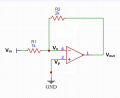Let's first analyze this using the "differential-amp-with-high-gain" model. We will assume that there is no current flowing into or out of the inputs.

Vp = 0 V

We will assume that there is no current flowing into or out of the inputs. This means that any current flowing from Vin into R1 will flow up through R2 and then to Vout (and into the output of the opamp).

So what is Vn?

If the current described before is Iin, we have

Iin = (Vin - Vout)/(R1+R2)

That makes

Vn = Vin - Iin*R1 = Vin - (Vin - Vout) * (R1/(R1+R2))

Vn = Vin*(R2/(R1+R2)) + Vout*(R1/(R1+R2)

Now we have our diff-amp equation

Vout = (Vp - Vn)*K

For us, this becomes

Vout = -Vn*K

Vout = -[Vin*(R2/(R1+R2)) + Vout*(R1/(R1+R2)]*K

Solving for Vout, we get

Vout * [1 + (R1/(R1+R2)*K] = -Vin*(R2/(R1+R2))*K

A bit of algebra, and you get:

Vout = -Vin*(R2*K)/(R1+R2+R1*K)

Assuming K = 100,000 and using R1 = 1 kΩ, and R2 = 2 kΩ, this works out to

Vout = -1.99994 * Vin

If Vin = 1 V, then

Vout = -1.99994 V

What is Vn, for this input? Simple, since Vp = 0, Vn = Vout/K, or

Vn = -19.9994 µV

As you can see, there's a lot of math -- and therefore a lot of opportunity for math mistakes -- doing the analysis this way.

So let's use the "ideal opamp" model, which says that the gain is infinite.

IF the gain is infinite -- and IF the circuit is configured so that we have the necessary negative feedback -- then the output voltage will settle at whatever voltage it needs to be in order to make the two inputs be at the same voltage. This is known as a "virtual short", since while the voltages at both inputs is zero, no current can flow between them because, in reality, they are not physically shorted together.

For our circuit, that means that Vn = Vp = 0 V.

If Vn = 0 V, that means that

In = Vin / R1

Since that current has to flow through R2 to get to Vout, that means that

Vout = -In*R2 = -(R2/R1)*Vin

For our values of the resistors, that means that

Vout = -2*Vin

Done!

While this is not exact, consider that it is off by only 0.003% and our resistors are unlikely to be closer than 1% to their nominal values, which completely swamps whatever error our use of the ideal opamp approach results in.

Now, you might notice that for Vin = 1 V, that Vout is -2 V.

If our opamp is powered by 0 V and 3.3 V supplies, it can't produce an output of -2 V. It will end up outputting the lowest voltage that it can and then stall there.

This is a common mistake that people make -- they ignore the limitations placed on the input/output voltages by the supplies.

A common guide is to not allow the inputs or the outputs to get within 3 V of either supply. If you need to get closer, then you need to spend time identifying an opamp that can do so (and plenty of them exist).

So, for this circuit, let's say that your input signals range from 0 V to 5 V. This means that Vout will range from 0 V to -10 V.

So your supplies should be no more than -3 V for the negative supply and no less than +13 V for the positive supplies. For a variety of reasons, it is customary to run opamps from symmetric supplies when practical. So a lot of people would choose to use ±15 V supplies or might go with ±12 V supplies, making sure they used an opamp that performed adequately within 2 V of the rails.

•xox and JosXD

#### JosXD

Joined Mar 16, 2022
33
in your unnamed circuit with feedback ("C"?), one input is at 0V. so the other is supposed to be also at 0V (or try to get there).
this means current through R1 is 1mA. but since input impedenace is high, the only way for current is through R2 to output. to keep the input at 0V (to match other input), output would need to go to -2V. but... that is impossible with that supply - any point in the circuit can only be between 0V and 3.3V. so output will try to go to -2V but the lowest it can get to is 0V. so the current from 1V (before R1) will not really be 1mA, it will be lower since (1V-0V)/(R1+R2)=1V/3k=0.33mA and the input is not really at 0V, more like 0.66V.

Oh man, that explanation is helping me a lot, here is another picture, can the 0.33mA go throught Vo to -V source or can't?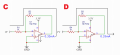#### WBahn

Joined Mar 31, 2012
29,164
So there is not short between -V and Vo, because between them there are transistors and those cause the small voltage drop?
You are still trying to think in terms of the opamp trying to create a short between the output and one of the supplies.

Forget this.

There is a output driver stage in the opamp that, combined with all of the other circuitry, is trying to make the output equal to the real-high open-loop gain times the differential input voltage. It does this by either sourcing current from the positive supply or sinking current to the negative supply. It sources or sinks just enough to make that output voltage happen. If it isn't sourcing/sinking enough, it will turn the transistors on a bit harder. If it is sourcing/sinking too much, it will turn the transistor on a bit less.

•panic mode and Ya’akov

#### WBahn

Joined Mar 31, 2012
29,164

Oh man, that explanation is helping me a lot, here is another picture, can the 0.33mA go throught Vo to -V source or can't?
View attachment 295924
The opamp will sink current as hard as it can to make the output go as low as it can. Assuming you have an opamp with rail-to-rail outputs, then the output will end up very close to 0 V and the 0.33 mA will be sunk to the negative supply (GND in this case).

But this is also assuming that the opamp will work correctly with the inputs so close to the supply voltage (you have the non-inverting input tied to the negative supply). Many opamps are NOT guaranteed to operate if either of the inputs is closer to either rail than a certain amount. This is usually referred to as the input common-mode range.

•panic mode and JosXD

#### JosXD

Joined Mar 16, 2022
33
There's LOTS of videos out there made by people that don't have a clue what they are talking about.

Forget this notion of the opamp shorting the output to one of the supplies. Just forget it.

Here's about the simplest description of an opamp that I can think of that is useful in understanding the basics of how it works:

It is a differential amplifier with a very large gain.

That's it -- for a first-order and often more-than-sufficient level of detail. Lots of other considerations exist, but until you get a handle on this one, the others don't matter.

Vout = (V+ - V-)*K

I read it all, very useful, will try to do some calculations.

#### crutschow

Joined Mar 14, 2008
32,903
•